JMSLTM Numerical Library 7.2.0
com.imsl.stat

## Class KolmogorovOneSample

• All Implemented Interfaces:
Serializable

```public class KolmogorovOneSample
extends Object
implements Serializable```
The class `KolmogorovOneSample` performs a Kolmogorov-Smirnov goodness-of-fit test in one sample.

The hypotheses tested follow: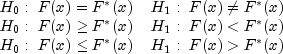where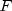is the cumulative distribution function (CDF) of the random variable, and the theoretical cdf,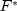, is specified via the user-supplied function cdf. Let n be the number of observations minus the number of missing observations. The test statistics for both one-sided alternatives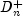and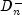and the two-sided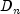alternative are computed as well as an asymptotic z-score and p-values associated with the one-sided and two-sided hypotheses. For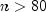, asymptotic p-values are used (see Gibbons 1971). For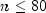, exact one-sided p-values are computed according to a method given by Conover (1980, page 350). An approximate two-sided test p-value is obtained as twice the one-sided p-value. The approximation is very close for one-sided p-values less than 0.10 and becomes very bad as the one-sided p-values get larger.

The theoretical CDF is assumed to be continuous. If the CDF is not continuous, the statistics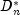will not be computed correctly.

Estimation of parameters in the theoretical CDF from the sample data will tend to make the p-values associated with the test statistics too liberal. The empirical CDF will tend to be closer to the theoretical CDF than it should be.

No attempt is made to check that all points in the sample are in the support of the theoretical CDF. If all sample points are not in the support of the CDF, the null hypothesis must be rejected.

Example, Serialized Form
• ### Constructor Summary

Constructors
Constructor and Description
```KolmogorovOneSample(CdfFunction cdf, double[] x)```
Constructs a one sample Kolmogorov-Smirnov goodness-of-fit test.
• ### Method Summary

Methods
Modifier and Type Method and Description
`double` `getMaximumDifference()`
Returns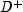, the maximum difference between the theoretical and empirical CDF's.
`double` `getMinimumDifference()`
Returns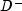, the minimum difference between the theoretical and empirical CDF's.
`int` `getNumberMissing()`
Returns the number of missing values in the data.
`int` `getNumberOfTies()`
Returns the number of ties in the data.
`double` `getOneSidedPValue()`
Probability of the statistic exceeding D under the null hypothesis of equality and against the one-sided alternative.
`double` `getTestStatistic()`
Returns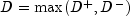.
`double` `getTwoSidedPValue()`
Probability of the statistic exceeding D under the null hypothesis of equality and against the two-sided alternative.
`double` `getZ()`
Returns the normalized D statistic without the continuity correction applied.
• ### Methods inherited from class java.lang.Object

`clone, equals, finalize, getClass, hashCode, notify, notifyAll, toString, wait, wait, wait`
• ### Constructor Detail

• #### KolmogorovOneSample

```public KolmogorovOneSample(CdfFunction cdf,
double[] x)```
Constructs a one sample Kolmogorov-Smirnov goodness-of-fit test.
Parameters:
`cdf` - is the cdf function,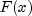. If must be non-decreasing and its value must be in [0, 1].
`x` - is a `double` array containing the observations.
• ### Method Detail

• #### getMaximumDifference

`public double getMaximumDifference()`
Returns, the maximum difference between the theoretical and empirical CDF's.
Returns:
The value.
• #### getMinimumDifference

`public double getMinimumDifference()`
Returns, the minimum difference between the theoretical and empirical CDF's.
Returns:
The value.
• #### getNumberMissing

`public int getNumberMissing()`
Returns the number of missing values in the data.
Returns:
The number of missing values.
• #### getNumberOfTies

`public int getNumberOfTies()`
Returns the number of ties in the data.
Returns:
the number of ties in the data
• #### getOneSidedPValue

`public double getOneSidedPValue()`
Probability of the statistic exceeding D under the null hypothesis of equality and against the one-sided alternative. An exact probability is computed if the number of observation is less than or equal to 80, otherwise an approximate probability is computed.
Returns:
the one-sided probability.
• #### getTestStatistic

`public double getTestStatistic()`
Returns.
Returns:
The value D.
• #### getTwoSidedPValue

`public double getTwoSidedPValue()`
Probability of the statistic exceeding D under the null hypothesis of equality and against the two-sided alternative. This probability is twice the probability,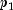, reported by `getOneSidedPValue`, (or 1.0 if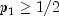). This approximation is nearly exact when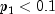.
Returns:
the two-sided probability.
• #### getZ

`public double getZ()`
Returns the normalized D statistic without the continuity correction applied.
Returns:
the value Z
JMSLTM Numerical Library 7.2.0